1

# 0.導讀

https://www.w3schools.com/html/default.asp

https://github.com/myyang/mysite

# 1.Python第一章就Go

## 1.2 Python的下載與安裝

### CPython直譯器

Python官方網站: https://www.python.org/

``````python --version
``````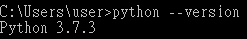### 設定環境變數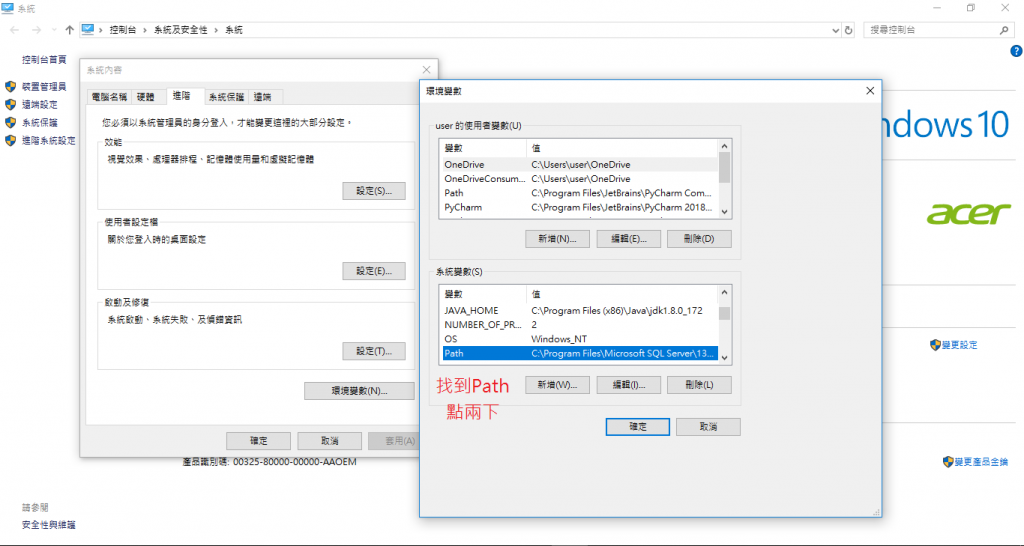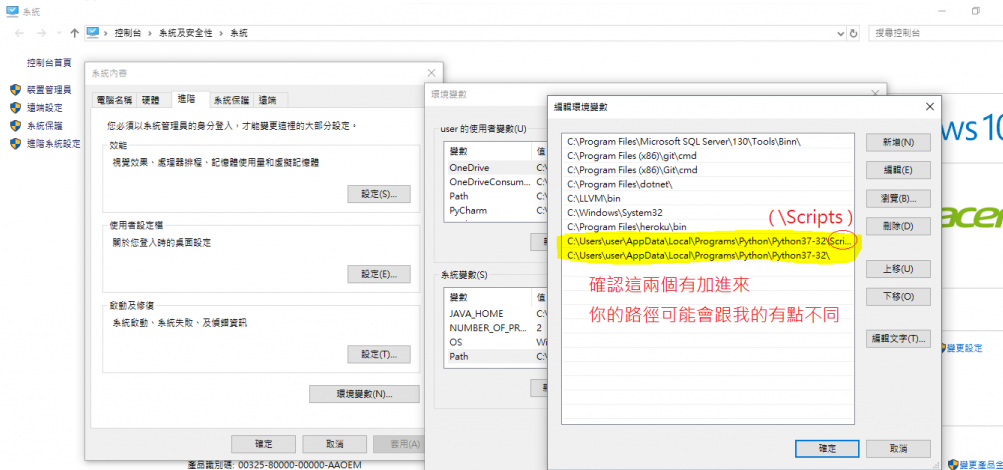### 1.3.1 進入 Python shell

``````python
``````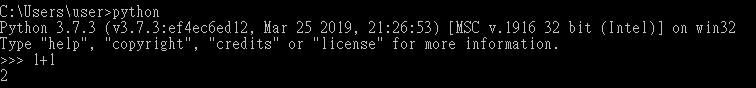### 1.3.2 Python的文件與求助

``````help()
``````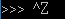### 1.3.7 撰寫多個運算

``````cd 要進入的目錄
``````

``````cd..
``````

``````md 要建立的目錄名稱
``````

``````mkdir 要建立的目錄名稱
``````

``````D:
``````

``````python test.py
``````

``````print result
``````

``````print(result)
``````

## 1.4 資料型態

### 1.4.1 整數(Integer)與浮點數(Float)

`/` 這裡要注意一下在python2.X中，如果`/`兩邊為整數，會變為取商；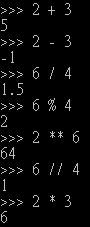1.2乘以10的2次方=120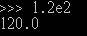#### doc string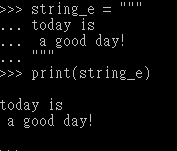### 1.4.3 字串(String)

#### 字串的格式化

``````'My name is {0}, I am {1} years old.'.format('dokelung', 27)
``````

``````'My name is {name}, I am {age} years old.'.format(name='dokelung', age=27)
``````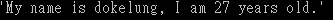#### 使用中文時的編碼問題

``````# -*- coding: utf-8 -*-
``````

List中的元素為可變動的

### 1.4.5 元組(Tuple)

Tuple中的元素為不可變動的，如果嘗試去更動，將會出現TypeError的錯誤

``````t = (1,)
``````

## 1.5 運算

### 1.5.6 身分運算

`==`只能用來判斷兩個資料的"值"相等而已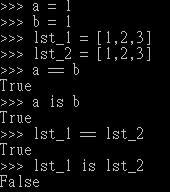## 1.6 流程控制

### 1.6.1 有條件的運算

``````string = '現在是春季' if 3 <= month <= 5 else '現在不是春季'
``````

### 1.6.2 重複的運算

#### list Comprehension

``````lst = [1, 2, 3, 4, 5]
``````

``````lst_sq = []
for num in lst:
lst_sq.append(num**2)
``````

``````lst_sq = [num**2 for num in lst]
``````

``````lst_sq = [num**2 for num in lst if num%2==0]
``````

#### dictionary comprehension

``````scores = [88, 90, 100, 65, 78]
score_dic = {student_id:score for student_id, score in enumerate(scores)}
``````

enumerate()這個函式和for搭配可以把元素的索引跟元素取出來用兩個變數迭代

## 1.7 輸入與輸出

print預設會輸出換行，如果不想換行必須這樣寫
(python2.X)

``````print 1,
print 2,
print 3
``````

(python3.X)

``````print(1, end='')
``````

### 檔案讀寫

``````Hello world
Today is a good day!
``````

open函式預設是用來"讀取"檔案

``````f = open('test_file')
f.close()
``````

output:

``````Hello world

Today is a good day!
``````

``````f = open('test_file')
f.close()
``````

output:

``````Hello world
Today is a good day!
``````

``````f = open('test_file')
f.seek(0)
f.close()
``````

seek(0)代表回到第0行(第1行)
output:

``````Hello world
Today is a good day!
Hello world
``````

``````f = open('test_file')
for line in f:
print(line.strip())
f.close()
``````

python的"環境管理器"功能，透過`with as`簡化我們的檔案讀寫

``````with open('test_file') as f:
for line in f:
print(line.strip())
``````

(python2.X)

``````lst = ['第一行', '第二行']
with open('test_file', 'w') as f:
for line in lst:
print >> f, line
``````

(python3.X)

``````lst = ['第一行', '第二行']
with open('test_file', 'w') as f:
for line in lst:
print(line, file=f)
``````

## 1.8 例外與捕捉

``````3 4
1 3

20 19
%%%
88 7
1 2 3
0
``````

``````7
4
39
95

``````

``````with open('test_file') as f:
for line in f:
try:
a, b = line.strip().split() # 這種寫法稱為unpack
print(int(a)+int(b))
except ValueError:
pass # 空運算
``````

## 1.9 函式

bool()

list()

set()

### 全部或任何

``````pass = [True, True, True, False, False]
``````

``````all_pass = True
for s in pass:
if s==False:
all_pass = False
if all_pass:
print('歐趴')
else:
print('有人不及格')
``````

``````if all(pass):
print('歐趴')
else:
print('有人不及格')
``````

### 1.9.2 自定義函式

#### 遞迴

``````lst = [1, 2, [3, 4, 5], 6, [7, [8,9]]]
``````

``````def print_list(lst):
for item in lst:
if isinstance(item, list): # 如果item是list型態就回傳True，否則回傳False
print_list(item)
else:
print(item)
``````

### 1.9.3 綴星運算式

``````def add(a, b, c, d):
return a+b+c+d
``````

``````lst = [1, 2, 3, 4]
``````

``````def add(*tup): # 將傳進來的所有元素組成一個tuple
return sum(tup)
``````

``````def power(base, exp):
return base ** exp
dic = {'base':2, 'exp':3}
print(power(**dic)) # 拆解成base=2 exp=3
``````
``````def power(**dic):
return dic['base'] ** dic['exp']
print(power(base=2, exp=3)) # 形成字典 {'base':2, 'exp':3}
``````

### 1.9.5 閉包與裝飾器

``````def gen_power(base):
def power(exp): # 區域函式，power函式就是閉包
return base ** exp
return power
power2 = gen_power(2)
power3 = gen_power(3)
print(power2(3)) # 8
print(power3(2)) # 9
``````

#### 裝飾器

``````def print_result(func):
def modified_func(*args):
print(args)
print(func(*args))
return modified_func

@print_result
return sum(tup)

@print_result
def power(base, exp):
return base ** exp

power(2, 3) # 相當於 power = print_result(power)
``````

``````(1, 2, 3, 4, 5)
15
(2, 3)
8

``````

``````def print_result(head):
def decorator(func):
def modified_func(*args, **kwargs):
result = func(*args, **kwargs)
return modified_func
return decorator

``````result: 15